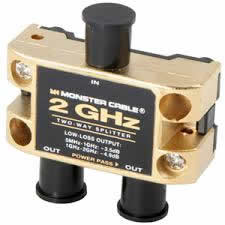# Difference between MHZ and GHZ

Key Difference: Mhz and Ghz both are used to denote frequency units. Mhz stands for megahertz (10^6 hertz) whereas Ghz stands for gigahertz (10^9 hertz).The hz in mhz and ghz stands for hertz. Hertz is the unit of measuring frequency. It is expressed as number of cycles occurring per second. Any phenomenon that has periodic variations can be expressed in terms of hertz. This unit is used in context to a lot of terms like alternating electric currents, electromagnetic waves and sound.Or we can also say that the SI unit of frequency is a frequency that is equal to one oscillation or cycle per second. The unit has a special name, the hertz (Hz). It is a SI unit which means that it is a part of International System of Units (based on metric system). One hertz simply refers to one cycle per second. A German physicist Heinrich Hertz made vital scientific contributions in the field of electromagnetism. In order to honour this scientist, hertz was established as the unit of frequency by the International Electro technical Commission and later was adopted by General Conference on Weights and Measures. Hertz is generally referred as hz. It is easy to write the unit in multiples of hertz.

Mhz and Ghz both are multiples of hertz denoting-Mhz stands for megahertz which is equivalent to 1000000 or 10^6 hertz

Ghz stands for gigahertz which is equivalent to 1000000000 or 10^9 hertz

This convention of writing Mhz and Ghz in place of 10^6 hertz and 10^9 hertz respectively, is easy to read, write and remember . Some other prefixes used with hertz are kilohertz (Khz), terahertz (Khz), petahertz (Phz) and many more.

Image Courtesy: mikepekula.com, govgroup.com